Symbol
ProblemBy ashat skoeld numbershould3\ $\left(\dfrac {3} {5}\right)b$ divided bo $9^{0t}\left(\dfrac {5} {3}\right)^{2}$ $-$ $n|m$
7th-9th grade
Other
Solution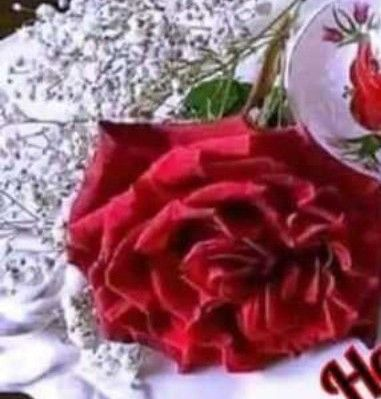Qanda teacher - harshit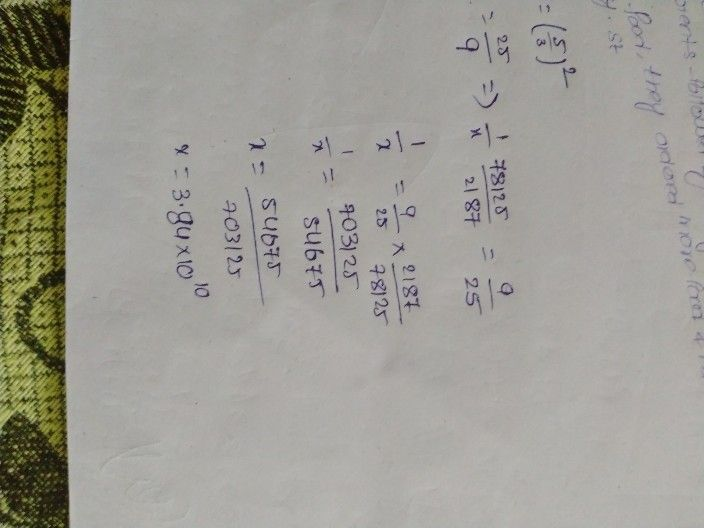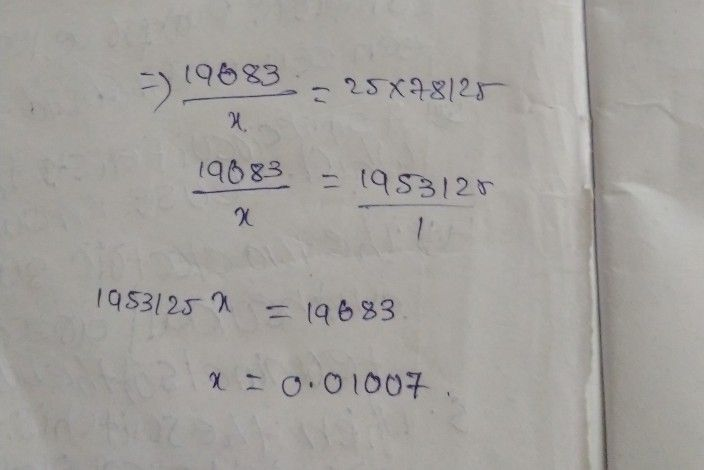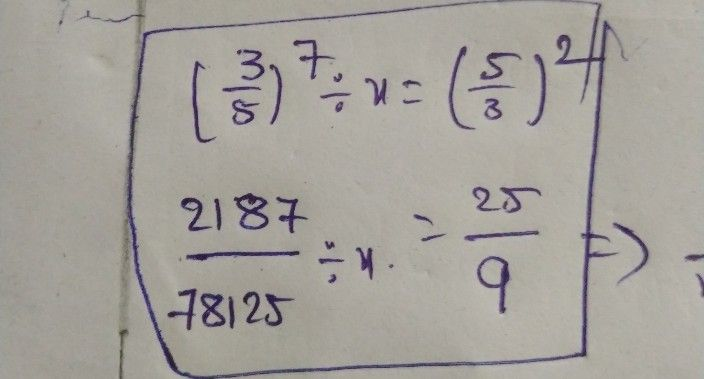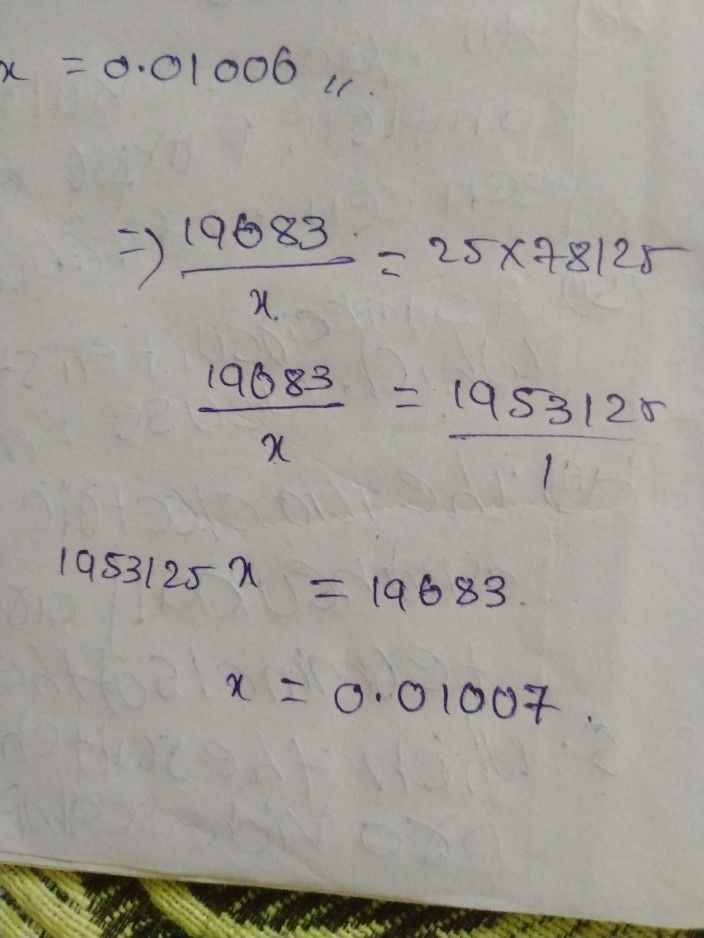delete first pic
last two pictures contain solutions